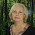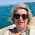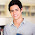## Wednesday, June 29, 2016

### 11 Ways to Teach Math with Google Drawings

Google Drawings is one of my favorite parts of the Google Drive suite, even though it seems to often get overlooked. Perhaps that is because Google itself hides the Drawings link under the “More” menu in Drive rather than letting it sit at the grown up table with Docs, Sheets, and Slides.

However, I think Drawings deserves much more attention especially in the classroom where it has so many educational uses. These include:

In addition to those ideas, one of my favorite uses for Google Drawings is teaching math. Drawings lends itself very well to mathematics with its shapes, rotations, tables, lines, and much more.

See below for 11 ideas for how to use Google Drawings to teach and learn math. In each case I have included a brief explanation of the idea, a Google Drawing template or example, and Common Core math standards addressed.

1) Lines of Symmetry

Students can create shapes or insert images, then add lines to show the lines of symmetry for those shapes.

Lines of Symmetry example - Google Drawing link

• CC.4.G.3 Draw and identify lines and angles, and classify shapes by properties of their lines and angles. Recognize a line of symmetry for a two-dimensional figure as a line across the figure such that the figure can be folded along the line into matching parts. Identify line-symmetric figures and draw lines of symmetry.

2) Partitioning Shapes

Students can create shapes and then add lines to partition the shapes into equal parts.

Splitting Rectangles example - Google Drawing link

• CC.1.G.3 Reason with shapes and their attributes. Partition circles and rectangles into two and four equal shares, describe the shares using the words halves, fourths, and quarters, and use the phrases half of, fourth of, and quarter of. Describe the whole as two of, or four of the shares. Understand for these examples that decomposing into more equal shares creates smaller shares.
• CC.2.G.3 Reason with shapes and their attributes. Partition circles and rectangles into two, three, or four equal shares, describe the shares using the words halves, thirds, half of, a third of, etc., and describe the whole as two halves, three thirds, four fourths. Recognize that equal shares of identical wholes need not have the same shape.
• CC.3.G.2 Reason with shapes and their attributes. Partition shapes into parts with equal areas. Express the area of each part as a unit fraction of the whole. For example, partition a shape into 4 parts with equal area, and describe the area of each part is 1/4 of the area of the shape.

3) Shapes

Students can create a picture by inserting a variety of required shapes, and can then add colors and other images to complete their picture. Students could then use Docs to explain which shapes they used in the picture and where the shapes are.

Shape Art Project example - Google Drawing link

• CC.K.G.5 Analyze, compare, create, and compose shapes. Model shapes in the world by building shapes from components (e.g., sticks and clay balls) and drawing shapes.
• CC.1.G.1 Reason with shapes and their attributes. Distinguish between defining attributes (e.g., triangles are closed and three-sided) versus non-defining attributes (e.g., color, orientation, overall size); for a wide variety of shapes; build and draw shapes to possess defining attributes.
• CC.1.G.2 Reason with shapes and their attributes. Compose two-dimensional shapes (rectangles, squares, trapezoids, triangles, half-circles, and quarter-circles) or three-dimensional shapes (cubes, right rectangular prisms, right circular cones, and right circular cylinders) to create a composite shape, and compose new shapes from the composite shape. (Students do not need to learn formal names such as “right rectangular prism.”)
• CC.2.G.1 Reason with shapes and their attributes. Recognize and draw shapes having specified attributes, such as a given number of angles or a given number of equal faces.  Identify triangles, quadrilaterals, pentagons, hexagons, and cubes. (Sizes are compared directly or visually, not compared by measuring.)
• CC.3.G.1 Reason with shapes and their attributes. Understand that shapes in different categories (e.g., rhombuses, rectangles, and others) may share attributes (e.g., having four sides), and that the shared attributes can define a larger category (e.g., quadrilaterals). Recognize rhombuses, rectangles, and squares as examples of quadrilaterals, and draw examples of quadrilaterals that do not belong to any of these subcategories.
• CC.4.G.2 Draw and identify lines and angles, and classify shapes by properties of their lines and angles. Classify two-dimensional figures based on the presence or absence of parallel or perpendicular lines, or the presence or absence of angles of a specified size. Recognize right triangles as a category, and identify right triangles.
• CC.7.G.2 Draw, construct, and describe geometrical figures and describe the relationships between them.  Draw (freehand, with ruler and protractor, and with technology) geometric shapes with given conditions. Focus on constructing triangles from three measures of angles or sides, noticing when the conditions determine a unique triangle, more than one triangle, or no triangle.

4) Fractions

Students can create their own country flag using a variety of colored shapes, then they can use Docs to explain which fractional part of the whole each color represents.

Fraction Flag example - Google Drawing link

• CC.3.NF.1 Develop understanding of fractions as numbers. Understand a fraction 1/b as the quantity formed by 1 part when a whole is partitioned into b equal parts; understand a fraction a/b as the quantity formed by a parts of size 1/b.  (Grade 3 expectations in this domain are limited to fractions with denominators 2, 3, 4, 6, and 8.)
• CC.3.NF.3b Recognize and generate simple equivalent fractions (e.g., 1/2 = 2/4, 4/6 = 2/3), Explain why the fractions are equivalent, e.g., by using a visual fraction model. (Grade 3 expectations in this domain are limited to fractions with denominators 2, 3, 4, 6, and 8.)

5) Pictographs and Line Plots

Students can insert a table, text, and images into a Drawing to create a pictograph or a line plot

• CC.2.MD.9 Represent and interpret data. Generate measurement data by measuring lengths of several objects to the nearest whole unit, or by making repeated measurements of the same object. Show the measurements by making a line plot, where the horizontal scale is marked off in whole-number units.
• CC.2.MD.10 Represent and interpret data. Draw a picture graph and a bar graph (with single-unit scale) to represent a data set with up to four categories. Solve simple put-together, take-apart, and compare problems  using information presented in a bar graph.
• CC.3.MD.3 Represent and interpret data. Draw a scaled picture graph and a scaled bar graph to represent a data set with several categories. Solve one- and two-step “how many more” and “how many less” problems using information presented in scaled bar graphs. For example, draw a bar graph in which each square in the bar graph might represent 5 pets.
• CC.3.MD.4 Represent and interpret data. Generate measurement data by measuring lengths using rulers marked with halves and fourths of an inch. Show the data by making a line plot, where the horizontal scale is marked off in appropriate units—whole numbers, halves, or quarters.
• CC.4.MD.4 Represent and interpret data. Make a line plot to display a data set of measurements in fractions of a unit (1/2, 1/4, 1/8). Solve problems involving addition and subtraction of fractions by using information presented in line plots. For example, from a line plot find and interpret the difference in length between the longest and shortest specimens in an insect collection.
• CC.5.MD.2 Represent and interpret data. Make a line plot to display a data set of measurements in fractions of a unit (1/2, 1/4, 1/8). Use operations on fractions for this grade to solve problems involving information presented in line plots. For example, given different measurements of liquid in identical beakers, find the amount of liquid each beaker would contain if the total amount in all the beakers were redistributed equally.

6) Congruent Shapes

Students can use the tools in Drawings to move (translate), flip (reflection), and rotate shapes to determine congruency.

Congruent Shapes example - Google Drawing link

• CC.8.G.1 Understand congruence and similarity using physical models, transparencies, or geometry software. Verify experimentally the properties of rotations, reflections, and translations: a. Lines are taken to lines, and line segments to line segments of the same length.b. Angles are taken to angles of the same measure.c. Parallel lines are taken to parallel lines.
• CC.8.G.2 Understand congruence and similarity using physical models, transparencies, or geometry software.  Understand that a two-dimensional figure is congruent to another if the second can be obtained from the first by a sequence of rotations, reflections, and translations; given two congruent figures, describe a sequence that exhibits the congruence between them.
• CC.9-12.G.CO.3 Experiment with transformations in the plane. Given a rectangle, parallelogram, trapezoid, or regular polygon, describe the rotations and reflections that carry it onto itself.
• CC.9-12.G.CO.5 Experiment with transformations in the plane. Given a geometric figure and a rotation, reflection, or translation, draw the transformed figure using, e.g., graph paper, tracing paper, or geometry software. Specify a sequence of transformations that will carry a given figure onto another.
• CC.9-12.G.CO.6 Understand congruence in terms of rigid motions. Use geometric descriptions of rigid motions to transform figures and to predict the effect of a given rigid motion on a given figure; given two figures, use the definition of congruence in terms of rigid motions to decide if they are congruent.

7) Similar Shapes

Students can use the tools in Drawings to move (translate), flip (reflection), rotate, and dilate (resize) shapes to determine similarity.

Similar Shapes example - Google Drawing link

• CC.8.G.4 Understand congruence and similarity using physical models, transparencies, or geometry software.  Understand that a two-dimensional figure is similar to another if the second can be obtained from the first by a sequence of rotations, reflections, translations, and dilations; given two similar two-dimensional figures, describe a sequence that exhibits the similarity between them.
• CC.9-12.G.SRT.2 Understand similarity in terms of similarity transformations. Given two figures, use the definition of similarity in terms of similarity transformations to decide if they are similar; explain using similarity transformations the meaning of similarity for triangles as the equality of all corresponding pairs of angles and the proportionality of all corresponding pairs of sides.

8) Angles of a Triangle

Students can copy, paste, and rotate copies of a triangle to demonstrate that the three angles of a triangle always add up to 180 degrees.

Angles of a Triangle example - Google Drawing link

• CC.9-12.G.CO.10 Prove geometric theorems. Prove theorems about triangles. Theorems include: measures of interior angles of a triangle sum to 180 degrees; base angles of isosceles triangles are congruent; the segment joining midpoints of two sides of a triangle is parallel to the third side and half the length; the medians of a triangle meet at a point.

9) Sorting Objects

Students can drag and drop objects into three categories based on a variety of characteristics including shape, color, and size.

3 Category Sorter - Shapes - Google Drawing link
3 Category Sorter - Colors - Google Drawing link
3 Category Sorter - Sizes - Google Drawing link
(more graphic organizers - Google Drive folder link )

• CC.K.MD.3 Classify objects and count the number of objects in each category. Classify objects into given categories; count the numbers of objects in each category and sort the categories by count. (Limit category counts to be less than or equal to 10.)
• CC.1.MD.4 Represent and interpret data. Organize, represent, and interpret data with up to three categories; ask and answer questions about the total number of data points, how many in each category, and how many more or less are in one category than in another.

Students can represent an integer addition problem by using and combining colored chips that model the positive and negative number being added.

• CC.6.NS.5 Apply and extend previous understandings of numbers to the system of rational numbers. Understand that positive and negative numbers are used together to describe quantities having opposite directions or values (e.g., temperature above/below zero, elevation above/below sea level, debits/credits, positive/negative electric charge); use positive and negative numbers to represent quantities in real-world contexts, explaining the meaning of 0 in each situation.
• CC.6.EE.7 Reason about and solve one-variable equations and inequalities. Solve real-world and mathematical problems by writing and solving equations of the form x + p = q and px = q for cases in which p, q and x are all nonnegative rational numbers.
• CC.7.NS.1b Understand p + q as the number located a distance |q| from p, in the positive or negative direction depending on whether q is positive or negative. Show that a number and its opposite have a sum of 0 (are additive inverses). Interpret sums of rational numbers by describing real-world contexts.

11) Algebraic Expressions

Students can copy and paste the algebra tile shapes in the template to multiply algebraic expressions.

Algebra Tiles Template - Google Drawing link

• CC.6.EE.3 Apply and extend previous understandings of arithmetic to algebraic expressions.  Apply the properties of operations to generate equivalent expressions. For example, apply the distributive property to the expression 3(2 + x) to produce the equivalent expression 6 + 3x; apply the distributive property to the expression 24x + 18y to produce the equivalent expression 6 (4x + 3y); apply properties of operations to y + y + y to produce the equivalent expression 3y.
• CC.9-12.A.APR.1 Perform arithmetic operations on polynomials. Understand that polynomials form a system analogous to the integers, namely, they are closed under the operations of addition, subtraction, and multiplication; add, subtract, and multiply polynomials.

More Resources

For more resources, see a detailed overview of each of these ideas, plus several more, in my one-hour training video below, as well as additional guides and documents.

Post by Eric Curts. Connect with Eric on Twitter at twitter.com/ericcurts and on Google+ at plus.google.com/+EricCurts1

1.Eric,
Thank you for the great ideas. I have used Google Drawings for social studies before. I am teaching math this year and may use some of your ideas. Thank you!

2.Great stuff Eric!!! Check out how I use gDrawings for formative assessments in the math classroom: https://www.youtube.com/watch?v=wGhDCjWsLjc

3.Во вкладке Фигуры есть цилиндр и куб. Маловато. Конус и пирамиду надо составлять из плоских фигур.

4.Thank you for the math ideas. We are working on shapes now in third grade and these are great ideas to get students thinking. Flipping the object and getting it to match is not easy either!.

5.If you are looking for some method do algebra online you first of all need to read this blog

6.Education is also a reason for the growth in your other extracurricular activities from sports to dramas, debates, elocution, athletics etc. Hence, it completes a man and helps him in attainment of skills necessary to be an all rounder.mathematics research statement

7.This is how the total number of data points, how many in each category, and how many more or less are in one category than in another with my site.

8.Cool ideas! For algebra 2, I used GDrawings for transformations of functions.